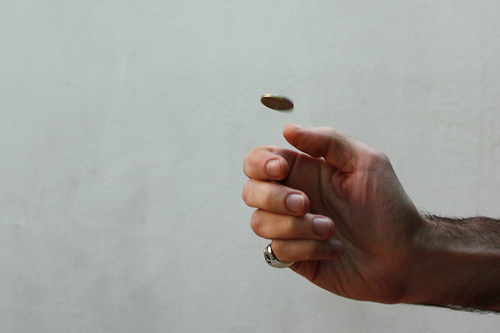# Questioning probability – Controlled probability theory

### Questioning the premise

We have been taught concepts of probability theory since young. A common probability theory statement goes like this:

“If we toss a fair coin, there are two equally likely outcomes: a head, or a tail.” This would imply that both the outcomes have 50% chance of happening. However, I would like to question this premise that chance of each outcome is 50%, i.e. a predefined constant.

### Proposing an alternative theory

I propose that the probability can be controlled, given a fair environment (in this case a fair coin is used).

There are three methods that the control(manipulation) can be achieved:

#### 1. The observable physical parameters(such as wind speed or humidity of air) can be manipulated, without being noticed by ordinary human beings.

In this method, the change in physical parameters can be very minor. Hence it would appear to ordinary human beings that the event occurs in a fair environment, but in actual fact the parameters have been manipulated to favour a certain outcome.

#### 2. The measurable physics constants(such as gravity or speed or light) can be manipulated, without being noticed by ordinary human beings.

Similarly in this method, the manipulation can be done in a subtle manner such that the event appears to be fair but in actual fact the slight change of physics constants can be controlled to favour a certain outcome.

#### 3. There exist non-observable parameters that can be manipulated, which have impact on observable events.

If this is true, it would be impossible for someone who has no knowledge of such parameters to know that manipulation is carried out, hence the event would appear to be fair. This would also imply that our knowledge about the observables is not complete.

It should be noted that although the nature of the three methods are different, they are not mutually exclusive, i.e. they can be combined to controlled an event.

### (Attempted) Proof of the theory

The proof would involve proving the three proposed methods to control the event:

#### 1. On controlling the observable physical parameters:

This method can be proved in the future. It is already theoretically possible to control these parameters. The only reason that this may be impractical is that ordinary human beings lack the abilities to interpret and manipulate such parameters. Hence, as soon as we can develop such abilities through evolution or artificial engineering, and we are able to take advantage of that to control the outcome of events, we can prove that this way is valid and practical.

#### 2.On controlling the measurable physics constants:

This method is hard to prove.  Currently we lack equipments to detect minor change in physics constants. In fact, we cannot even accurately measure these constants due to Heisenberg’s Uncertainty Principle.

#### 3. On controlling the non-observable parameters:

This method is even harder to prove. It would require the knowledge of non-observable parameters to prove that such way exists. Even if the proof is done, it would be impossible for ordinary human beings without the required knowledge to comprehend and agree upon.

### Implications of the theory

The implications, if the theory is true, is beyond imagination. It would mean that everything based on probability and statistics theory would collapse. Essentially almost everything can be controlled by those who have such ability.

This is one of the many interesting/crazy/random ideas that I have, you can check out here for more of them.

I am planning to develop a game, using the some of the concepts in this theory, leave a comment if you are interested to find out more about it.

This is too scary to be true.

1.Konstantin says:
1.paradite says: